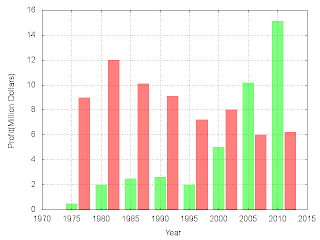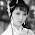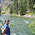## Saturday, September 17, 2011

### Plot histograms using boxes

Some one may ask:"There is histogram plot style in gnuplot, why plot it with boxes?" I would like to say there is some restriction on the built in histogram plot style, for example the x-axis is always using the row number, you can not make it using the coloumns in the data file.

The simplest case is that there is only one group of data to be plotted. In this case you just set the boxwidth to a proper value, for example 0.95, and plot it with boxes. Here is an example.
The data file is like this:
```1975    0.5     9.0
1980    2.0     12.0
1985    2.5     10.1
1990    2.6     9.1
1995    2.0     7.2
2000    5.0     8.0
2005    10.2    6.0
2010    15.1    6.2
```
The plotting script is like this:
```reset
set term png truecolor
set output "profit.png"
set xlabel "Year"
set ylabel "Profit(Million Dollars)"
set grid
set boxwidth 0.95 relative
set style fill transparent solid 0.5 noborder
plot "profit.dat" u 1:2 w boxes lc rgb"green" notitle
```
This example plot a graph like this one:Plot histogram using boxes with one group of data

When there is more than one group of data to plot, the boxwidth and gap between the boxes should be calculated carefully. We do it like this:
```reset
dx=5.
n=2
total_box_width_relative=0.75
gap_width_relative=0.1
d_width=(gap_width_relative+total_box_width_relative)*dx/2.
reset
set term png truecolor
set output "profit.png"
set xlabel "Year"
set ylabel "Profit(Million Dollars)"
set grid
set boxwidth total_box_width_relative/n relative
set style fill transparent solid 0.5 noborder
plot "profit.dat" u 1:2 w boxes lc rgb"green" notitle,\
"profit.dat" u (\$1+d_width):3 w boxes lc rgb"red" notitle
```
This time we get a histogram with two group of data like this:Plot histogram using boxes with more than one group of data

1.Your gnuplot blogs are very useful.

Could you explain what the parameters in these lines mean?

plot "profit.dat" u 1:2 w boxes lc rgb"green" notitle,\
"profit.dat" u (\$1+d_width):3 w boxes lc rgb"red" notitle

1.The full version of these two lines is:
plot "profit.dat" using 1:2 with boxes linecolor rgb"green" notitle,\
"profit.dat" using (\$1+d_width):3 with boxes linecolor rgb"red" notitle
where
"using 1:2" means plot the graph using the first and second column,
"with boxes" means plot with plotstyle---boxes,
linecolor rgb"green" means plot with green color
and at last "notitle" means the legend not plotted.

2.2.htmlResponseBytes 39842 66666
cssResponseBytes 109265 191911
imageResponseBytes 205179 222122
javascriptResponseBytes 468573 476666
otherResponseBytes 4326 4455

I want to create graph for this data, how can i modify your above code.

1.plot "file.dat" u 0:2 w boxes lc rgb"green" notitle
set xtics ("html" 0,"css" 1, "image" 2, "javascript" 3,"other" 4)

2.Or simply
plot "file.dat" u 0:2:xtic(1) w boxes lc rgb"green" notitle

3.Thank you for your post.

But I don't understand how that is going to work. What is meant by plotting 0:2:xtic(1)? Is it possible to create a column diagramm with more than one group of data?

Thank you in advance.

3.In addition, it allows you to plot lines over histogram-like boxes. You cannot do it if you use real histograms (as far as I know).
Thanks for the share !

4.5.very useful indeed

6.i want to plot the same histograms with fill pattern. please guide me.
i have 3 columns with 11 values in each column. i want to give different patterns to columns so that it can be readable in gray scale printed form.

1."set style fill pattern
plot sin(x) with boxes, cos(x) with boxes"
will plot sin(x) and cos(x) with different fill patterns. And your problem can be solved similarly.

7.And if you want to make this graph from the command line, what should be the syntax?

1.I am sorry! But I just did not get your meaning.

2.In Command Line : gnuplot file.p make sure u r in exact directory with dat file

8.I want to add my own data file but when i to it says no data to plot

9.What if we have more than 3 columns? Then how will we draw the graph

10.very useful blog post. Thank you.

11.Hi, I am new to gnuplot and would require your help. I want to plot a statistical histogram of 7 columns, having x at x-axis and frequency at Y axis. I have got the histogram using MATLAB, but I want to draw it using gnuplot. Example code of such histogram will be appriciated.
Thanks

12.How can I overlay a gaussian curve fitted on hinstogram plot?

13.Thats excellent!!

14.the post was really helpful, thank you